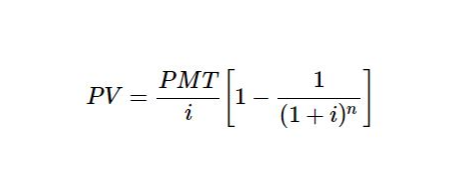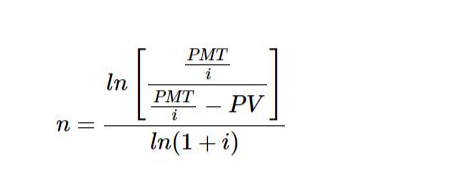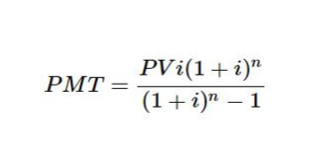# Loan Calculator

Loan payment calculator helps you to find the Loan Amount, Interest Rate, Number of Months, and Monthly Payment

## How to Use Duplichecker’s Loan Calculator?

The process of using this loan repayment calculator is free from any intricacies. This online facility provides great assistance in calculating different types of loans with a zero margin of error.

All you require is to follow the simple and easy instructions shared below to calculate the loan amount.

### Choose Calculation:

Our loan calculator has the capacity to handle various kinds of calculations and gives you accurate and flawless output.

Therefore, to start the loan calculation procedure, choose the calculation mode from the given options.

#### Find the Loan Amount:

1. Enter the loan amount in the given box.
2. Type the interest rate decided with the other party in the next field.
3. Add the number of months in which the loan amount will be repaid.
4. After entering all the values accurately, press the Calculate button.
5. The advanced loan calculator will process your inputs and gives you flawless results swiftly.
##### Formulation:

The simple loan calculator uses the loan equation formula to calculate the value,where

1. PV denotes the loan amount
2. PMT is used for monthly payment
3. i is the interest rate per month
4. n is the total number of months

Using this formulation, our loans calculator computes the loan amount on the basis of your entered values. No manual calculation is required to find the loan amount with our tool.

#### Find the Interest Rate:

1. Add the loan amount in the given field.
2. Insert the number of months decided in which you will repay the loan.
3. Enter the monthly installment amount in a given field.
4. Once you enter all the inputs accurately, press the Calculate button.
5. The facility will process your entered values and provides you with Interest Rate against your inputs.
##### Formulation:

Interest Rate = (Simple Interest × 100)/(Principal × Time)

The loan interest calculator makes no mistake in calculating the interest rate. Using this interest loan calculator, you can get the interest rate instantly by tapping only a few tabs.

#### Find the Number of Months:

In case you want to find the number of months to repay the total amount of the loan, follow the method given below.

1. First of all, enter the total loan amount.
2. Then, add the decided interest rate in the designated box.
3. After that, enter the monthly installment amount.
4. After adding all the inputs correctly, tap the Calculate button.
5. This monthly loan payment calculator will compute the entered values and show the accurate answer to your query.
##### Formulation:

The following equation will be used to find the number of months n;Calculating the number of months using this formula can take extensive time. But, the use of our loan calculator enables users to find the number of months to repay the loan within a few seconds.

#### Find the Monthly Payment

Find the monthly payment easily by following the method mentioned below straightaway

1. Insert the total loan amount.
2. Enter the Interest Rate.
3. Now, enter the number of months you decided to repay the loan
4. Hit the Calculate button after adding all the entries.
5. This facility will calculate the input and provides you with the exact monthly payment in no time.
##### Formulation:

Solve the following equation for PMT for finding the monthly payment:Facing problems in calculating monthly payments to settle the debt? Access our free loan payment calculator to find the accurate monthly loan payment right away.

## Loan Basics

The loan is the sum of an amount that an individual, company, or number of individuals collectively borrow from a bank or any other financial institution or person to manage or initiate events, businesses, or manage other tasks.

Below are the three primary aspects that must be analyzed adequately before taking a loan.

### Interest Rate

The interest rate is the additional amount that the borrower needs to pay to the lender besides the actual amount of the loan. The interest rate is basically a percentage of the principal amount loaned.

### Compounding Frequency

Compounding frequency is the total number of compounding periods in a single year. For instance, half-yearly compounding has a compounding frequency of 2.

### Loan Term

The loan term or terms of loans are the conditions decided between the lender and the borrower while giving the loan. The loan term includes the repayment period, the interest rate, and fees associated with the loan.

## Types of Loan Calculators

There are different types of loans individuals take to manage their unplanned events or operations.

### Auto Loan Calculator

Finding the monthly loan payment or overall loan value of the different types of autos becomes easy and simple to perform the task with the help of a loan rate calculator. Once you insert the values of your selected auto, like car model, model year, the total amount of car, down payment, loan duration, etc., in an online loan calculator, it will process it and give you an authentic answer within a few seconds.

### Mortgage Loan Calculator

This Loan calculator helps you estimate a monthly payment after entering the home price, down payment, interest rate, and loan term duration.

Similarly, you can check the loan amortization schedule and the amount of debt reduced with monthly principal and interest payments using an advanced loan calculator.

### Home loan calculator

A home equity loan also called a second mortgage, is mainly for homeowners who desire to borrow a specific portion of the equity to pay for home renovation, college fees, or any other expense. The home equity loan can be a one-time, repaid at a fixed rate, or lump-sum loan.

This free loan calculator enables you to find out the total money you can borrow on the basis of your credit score or loan-to-loan ratio, which is basically the difference between the actual worth of your home and how much you owe on it.

### Personal Loan Calculator

A personal loan is a lump sum loan that the borrower needs to repay at a fixed rate within a given time. The personal loan is considered a flexible loan as it can be used to consolidate debt, do a home renovation, pay school or college fees, pay for a wedding or any other event, buy any asset or make other purchases.

This loan calculator assists you in calculating the monthly payments on the basis of the total amount you borrow, the interest rate, and the time duration to repay the debt and your income.

### Student Loan Calculator

Students can often get a loan from either their governments or different private lenders to bear the expenses of their college or university. However, an established credit history is essential to stand eligible for the best loan.

The loan rate calculator will guide you about the duration in which you can pay off your loan and the amount of interest you need to bear with it. In addition, the assistance of this loan calculator enables students to set their saving goals accordingly.

This online loan calculator is an advanced utility that computes the payback amount and the total cost of a business loan. This loan amount calculator also asks for the fee to find out the true annual percentage rate of the loan. The true annual percentage or APR enables the borrowers to make a reliable assessment of the loan’s actual cost.

The use of a loan calculator is inevitable to determine the monthly repayments and credit charges for various kinds of loans, especially if you take a loan to finance your business, buy new equipment, or expand the workforce.

## FAQ’s

### What is a Loan Calculator?

The loan calculator is an advanced facility that helps you calculate the monthly payments on a loan. All you need is to enter the loan amount, the total number of months in which you repay the amount, and the interest rate and press the given tab.

### What Is the Average Interest Rate On a Loan?

As of Jan 18, 2023, the average personal loan interest rate is 10.56 percent. However, this can be higher or lower based on your specific financial circumstances.

The simple interest loan calculator helps you calculate the interest rate on a loan without requiring you to make any manual calculations.

### How Long to Pay Off Your Loan?

Use our advanced loan payoff calculator to find the number of months to pay off your loan. Simply enter the loan amount, interest rate, and monthly payment in the given boxes.

The facility will provide you with a total number of months required to repay the total debt.